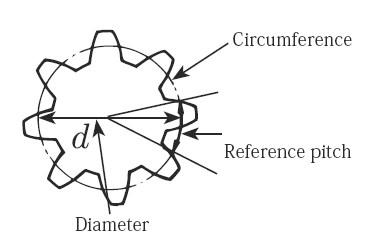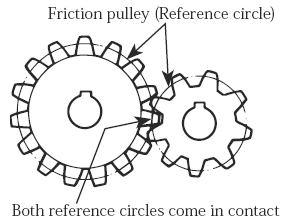Related Resources: calculators

### Spur Gear Calculator and Generator Download DXF, SVG or CSV Excel format files

Gear Design and Engineering

Spur Gear Calculator and Generator - Download DXF, SVG or CSV Excel format files

Simular: Spur Gear and assembly Builder

Spur Gear Generator is unitless: you may choose inches, cm or millimeters when importing your DXF file as you will have the same value for D/P as it is set above or as imported (SI or imperial nits). Gears in SVG are measured in pixels, which is the value multiplied with the scale (Pixel per Unit) as it is displayed on the right side.

"Module" is the unit of size that indicates how big or small a gear is. It is the ratio of the reference diameter of the gear divided by the number of teeth.

Thus:

m = d / z

Module = Reference Diameter * Number of teeth

NUmber of teeth:

z = d / m

Number of teeth = Reference Number / Module

Reference pitch :

p = π * ModuleReference Pitch = Circumference / Number of teeth = ( π d ) / zRelated: